Definition: area from Philip's Encyclopedia

Two-dimensional measurement of a plane figure or body (such as this page) given in square units, such as cm2 or m2. The area of a rectangle of sides a and b is ab; the areas of triangles and other polygons can be determined using trigonometry. Areas of curved figures and surfaces can be determined by integral calculus.

Summary Article: Lesson 10-7: Area
From Homework Helpers: Basic Math and Pre-Algebra

The amount of space that a closed polygon encloses is the area of the polygon. Area is measured in “square units.” In other words, the square is the basic unit for measuring area. Recall that a square is a quadrilateral that has four right angles and all four sides have the same length. The area of a square is defined to be the square of the length of one of its sides. A square with side length 1 inch has area 1 square inch, or 1 inch2. A square with side length 2 centimeters has an area of 4 square centimeters, or 4 centimeters2.

The area of a square is the motivation for calling the exponential expression a2, “a squared.” The area of a square of length a is “a squared,” or a2.

We use abbreviations for units of length. The most common units for length are inches, centimeters, and feet. Their abbreviations are “in,” “cm,” and “ft,” respectively. These abbreviations for units of length will help us abbreviate units of area. A square inch is abbreviated in2, a square centimeter is abbreviated cm2, and a square foot is abbreviated ft2.

Armed with a new ruler (the square unit, as in square inches) we can measure the area of other polygons besides a square. We will start with a rectangle and get more complicated. The area of a rectangle is the product of its length and its width. For example, a rectangle with length 4 inches and width 6 inches would enclose an area of 24 in2. A rectangle with length 10 feet and width 6 feet would enclose an area of 60 ft2.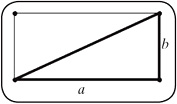Figure 10.14.

The area of a right triangle can be related to the area of a rectangle. You can think of a right triangle as one-half of a rectangle, as shown in Figure 10.14.

Notice that the width and length of the rectangle correspond to the two legs of the right triangle. The area of the rectangle is then the product of the lengths of the two legs of the triangle. Because two triangles make up one rectangle, it follows that the area of one triangle is one-half of the area of the rectangle. That gives us a formula for the area of a right triangle.

Example 1

Find the area of the polygons shown in Figure 10.15.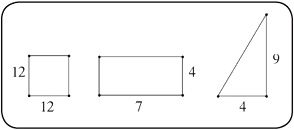Figure 10.15.

Solution: The first polygon is a square with side length 12. The area of the square is 144 unit2. The second polygon is a rectangle with length 4 and width 7. The area of the rectangle is 28 unit2. The third polygon is a right triangle with legs of length 9 and 4. The area of the right triangle is: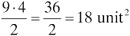In the previous example, I was able to find the area of a right triangle. Unfortunately, not every triangle is right. Before I can discuss the area of a run-of-the-mill triangle, I must define some terms. The base of a triangle is just one of the sides of the triangle singled out. Any side of a triangle can serve as the base. Once you specify the base of a triangle, there will be one vertex of the triangle that does not come in contact with the base. The distance from that vertex to the base is called the corresponding height of the triangle. There is no official height of a triangle. The height of a triangle depends on the side that serves as the base.

Now that we know about the base and the corresponding height of a triangle, we can find the area of a triangle. The area of a triangle is one-half the product of the length of the base and the corresponding height of the triangle. If b represents the length of the base and h represents the corresponding height of the triangle, the area enclosed by the triangle is given by this formula:

Example 2

Find the areas enclosed by the triangles shown in Figure 10.16.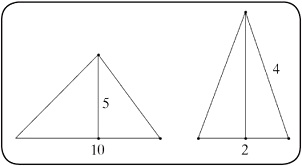Figure 10.16.

Solution: In the first triangle, the base has length 10 and the corresponding height is 5. The enclosed area is: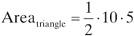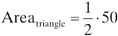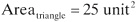The base of the second triangle has length 2 and the corresponding height is 4, so the enclosed area is: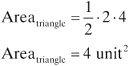Lesson 10-7 Review

Find the enclosed areas of the following polygons.

1. A rectangle with width 20 decimeters and length 2 decimeters.

2. A square with length 10 centimeters.

3. An isosceles right triangle with hypotenuse of length 8 inches.

4. A triangle with base 8 inches and corresponding height 4 inches.

5. A right triangle with one leg of length 3 feet and the hypotenuse of length 5 feet.Copyright 2011 © by Denise Szecsei

### Related Articles

##### Full text Article area
The Penguin Dictionary of Mathematics

A measure of a surface. For a rectangle, the area is the product of two adjacent sides. A triangle has an area equal to the product of half its...

##### Full text Article photon density
Stedman's Medical Dictionary

the number of counted events recorded in scintigraphy per square centimeter or per square inch of imaged area. count density

See more from Credo